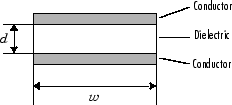txlineParallelPlate

Create parallel-plate transmission line

Description

Use the txlineParallelPlate object to create a parallel-plate transmission line. A cross-section of a parallel-plate transmission line is shown this figure. The physical characteristics of the parallel-plate transmission line include the plate width, w and the plate separation, d.Creation

Description

paralleltxline = txlineParallelPlate creates a parallel-plate transmission line object.

example

paralleltxline = txlineParallelPlate(Name,Value) sets properties using one or more name-value pairs. For example, txline = txlineParallelPlate('Separation',0.0046) creates a parallel-plate transmission line with a separation of 0.0046 meters.

Properties

expand all

Name of the parallel-plate transmission line, specified as a string scalar or a character vector.

Example: 'Name','parallelplate1'

Example: paralleltxline.Name = 'parallelplate1'

Data Types: char | string

Physical length of the transmission line, specified as a positive scalar in meters.

Example: 'LineLength',0.0200

Example: paralletxline.LineLength = 0.0200

Data Types: double

Physical width of the transmission line, specified as a positive scalar in meters.

Example: 'Width',0.00200

Example: paralleltxline.Width = 0.00200

Data Types: double

Thickness of dielectric, specified as a positive scalar in meters.

Example: 'Separation',0.007

Example: paralleltxline.Separation = 0.007

Data Types: double

Relative permeability of a dielectric, specified as a positive scalar.

The ratio of permeability of dielectric, μ, to the permeability in free space, μ0.

Example: 'MuR',1.5

Example: paralleltxline.MuR = 1.5

Data Types: double

Relative permittivity of the dielectric, specified as a positive scalar in Farad per meter (F/m).

Example: 'EpsilonR',3.3

Example: paralleltxline.EpsilonR = 3.3

Data Types: double

Loss angle tangent of the dielectric, specified as a nonnegative scalar.

Example: 'LossTangent',1

Example: paralleltxline.LossTangent = 1

Data Types: double

Conductivity of the conductor, specified as a positive scalar in Siemens per meter (S/m).

Example: 'SigmaCond',2

Example: paralleltxline.SigmaCond = 2

Data Types: double

Stub transmission line termination, specified as 'NotApplicable', 'Open', or 'Short'.

Example: 'Termination','Short'

Example: paralleltxline.Termination = 'Short'

Data Types: char

Type of stub, specified as 'NotAStub', 'Series', or 'Shunt'.

Example: 'StubMode','Series'

Example: paralleltxline.StubMode = 'Series'

Data Types: char

Number of input and output ports, specified as positive scalar.

Data Types: double

Terminals of coaxial transmission line, specified as a cell array of strings.

Data Types: char | string

Object Functions

 sparameters Calculate S-parameters for RF data, network, circuit, and matching network objects groupdelay Group delay of S-parameter object or RF filter object or RF Toolbox circuit object noisefigure Calculate noise figure of transmission lines, series RLC, and shunt RLC circuits getZ0 Calculate characteristic impedance with and without dispersion for transmission line circuit Circuit object clone Create copy of existing circuit element or circuit object

Examples

collapse all

Create a parallel-plate transmission line using these specifications:

• Width : 300 mm

• Separation between plates : 10 mm

• EpsilonR : 4.2 F/m

parallelplatetxline = txlineParallelPlate('Width',300e-3,'Separation',10e-3,'EpsilonR',4.2)
parallelplatetxline =
txlineParallelPlate: ParallelPlate element

Name: 'ParallelPlate'
Width: 0.3000
Separation: 0.0100
MuR: 1
EpsilonR: 4.2000
LossTangent: 0
SigmaCond: Inf
LineLength: 0.0100
Termination: 'NotApplicable'
StubMode: 'NotAStub'
NumPorts: 2
Terminals: {'p1+'  'p2+'  'p1-'  'p2-'}

Calculate the S-parameters of the transmission line at 6 GHz.

sparam = sparameters(parallelplatetxline,6e9);